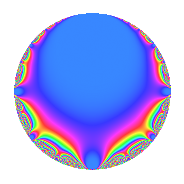# Properties

 Label 8.3.dLevel 8 Weight 3 Character orbit d Rep. character $$\chi_{8}(3,\cdot)$$ Character field $$\Q$$ Dimension 1 Newforms 1 Sturm bound 3 Trace bound 0

# Related objects

## Defining parameters

 Level: $$N$$ = $$8 = 2^{3}$$ Weight: $$k$$ = $$3$$ Character orbit: $$[\chi]$$ = 8.d (of order $$2$$ and degree $$1$$) Character conductor: $$\operatorname{cond}(\chi)$$ = $$8$$ Character field: $$\Q$$ Newforms: $$1$$ Sturm bound: $$3$$ Trace bound: $$0$$

## Dimensions

The following table gives the dimensions of various subspaces of $$M_{3}(8, [\chi])$$.

Total New Old
Modular forms 3 3 0
Cusp forms 1 1 0
Eisenstein series 2 2 0

## Trace form

 $$q$$ $$\mathstrut -\mathstrut 2q^{2}$$ $$\mathstrut -\mathstrut 2q^{3}$$ $$\mathstrut +\mathstrut 4q^{4}$$ $$\mathstrut +\mathstrut 4q^{6}$$ $$\mathstrut -\mathstrut 8q^{8}$$ $$\mathstrut -\mathstrut 5q^{9}$$ $$\mathstrut +\mathstrut O(q^{10})$$ $$q$$ $$\mathstrut -\mathstrut 2q^{2}$$ $$\mathstrut -\mathstrut 2q^{3}$$ $$\mathstrut +\mathstrut 4q^{4}$$ $$\mathstrut +\mathstrut 4q^{6}$$ $$\mathstrut -\mathstrut 8q^{8}$$ $$\mathstrut -\mathstrut 5q^{9}$$ $$\mathstrut +\mathstrut 14q^{11}$$ $$\mathstrut -\mathstrut 8q^{12}$$ $$\mathstrut +\mathstrut 16q^{16}$$ $$\mathstrut +\mathstrut 2q^{17}$$ $$\mathstrut +\mathstrut 10q^{18}$$ $$\mathstrut -\mathstrut 34q^{19}$$ $$\mathstrut -\mathstrut 28q^{22}$$ $$\mathstrut +\mathstrut 16q^{24}$$ $$\mathstrut +\mathstrut 25q^{25}$$ $$\mathstrut +\mathstrut 28q^{27}$$ $$\mathstrut -\mathstrut 32q^{32}$$ $$\mathstrut -\mathstrut 28q^{33}$$ $$\mathstrut -\mathstrut 4q^{34}$$ $$\mathstrut -\mathstrut 20q^{36}$$ $$\mathstrut +\mathstrut 68q^{38}$$ $$\mathstrut -\mathstrut 46q^{41}$$ $$\mathstrut +\mathstrut 14q^{43}$$ $$\mathstrut +\mathstrut 56q^{44}$$ $$\mathstrut -\mathstrut 32q^{48}$$ $$\mathstrut +\mathstrut 49q^{49}$$ $$\mathstrut -\mathstrut 50q^{50}$$ $$\mathstrut -\mathstrut 4q^{51}$$ $$\mathstrut -\mathstrut 56q^{54}$$ $$\mathstrut +\mathstrut 68q^{57}$$ $$\mathstrut -\mathstrut 82q^{59}$$ $$\mathstrut +\mathstrut 64q^{64}$$ $$\mathstrut +\mathstrut 56q^{66}$$ $$\mathstrut +\mathstrut 62q^{67}$$ $$\mathstrut +\mathstrut 8q^{68}$$ $$\mathstrut +\mathstrut 40q^{72}$$ $$\mathstrut -\mathstrut 142q^{73}$$ $$\mathstrut -\mathstrut 50q^{75}$$ $$\mathstrut -\mathstrut 136q^{76}$$ $$\mathstrut -\mathstrut 11q^{81}$$ $$\mathstrut +\mathstrut 92q^{82}$$ $$\mathstrut +\mathstrut 158q^{83}$$ $$\mathstrut -\mathstrut 28q^{86}$$ $$\mathstrut -\mathstrut 112q^{88}$$ $$\mathstrut +\mathstrut 146q^{89}$$ $$\mathstrut +\mathstrut 64q^{96}$$ $$\mathstrut -\mathstrut 94q^{97}$$ $$\mathstrut -\mathstrut 98q^{98}$$ $$\mathstrut -\mathstrut 70q^{99}$$ $$\mathstrut +\mathstrut O(q^{100})$$

## Decomposition of $$S_{3}^{\mathrm{new}}(8, [\chi])$$ into irreducible Hecke orbits

Label Dim. $$A$$ Field CM Traces $q$-expansion
$$a_2$$ $$a_3$$ $$a_5$$ $$a_7$$
8.3.d.a $$1$$ $$0.218$$ $$\Q$$ $$\Q(\sqrt{-2})$$ $$-2$$ $$-2$$ $$0$$ $$0$$ $$q-2q^{2}-2q^{3}+4q^{4}+4q^{6}-8q^{8}+\cdots$$